StatLect

# Joint probability mass function

The joint probability mass function is a function that completely characterizes the distribution of a discrete random vector. When evaluated at a given point, it gives the probability that the realization of the random vector will be equal to that point.## Synonyms and acronyms

The term joint probability function is often used as a synonym. Sometimes, the abbreviation joint pmf is used.

## Definition

The following is a formal definition.

Definition Letbe adiscrete random vector. Its joint probability mass function is a functionsuch that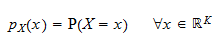whereis the probability that the random vectortakes the value.

This is a straightforward multi-variate generalization of the definition of the probability mass function of a discrete variable (uni-variate case).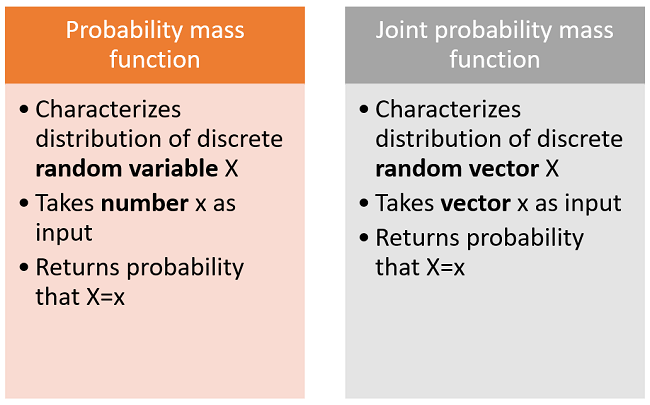## Example

Supposeis adiscrete random vector and that its support (the set of values it can take) is:If the three values have the same probability, then the joint probability mass function is: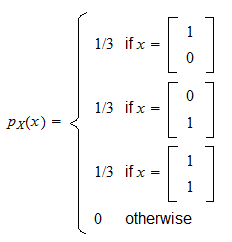When the two components ofare denoted byand, the joint pmf can also be written using the following alternative notation: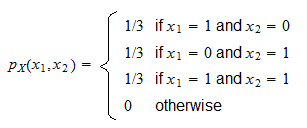## How to derive the marginals

The joint pmf can be used to derive the marginal probability mass functions of the single entries of the random vector.

Given, the marginal ofisIn order to get the entire marginal, we need to computeseparately for eachbelonging to the support of.

Each of the computations involves a sum over all the possible values of(i.e., over the support).

Similarly, the marginal ofis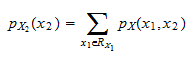### Example

Let us derive the marginal pmf offrom the joint pmf in the previous example.

The supports ofandareWe have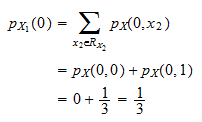and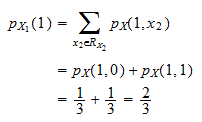Thus, the marginal probability mass function ofis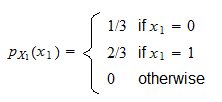## Joint pmf in tabular form

If a random vector has two entriesand, then its joint pmf can be written in tabular form:

• each row corresponds to one of the possible values of;

• each column corresponds to one of the possible values of;

• each cell contains the probability of a couple of values.

In the next example it will become clear why the tabular form is very convenient.

### Example of tabular form

Let us put in tabular form the joint pmf used in the previous examples.

x2=0x2=1Marginal of X1
x1=001/31/3
x1=11/31/32/3
Marginal of X21/32/3

We can easily obtain the marginals by summing the probabilities by column and by row.

## Conditional and joint pmf

The joint pmf can also be used to derive the conditional probability mass function of the single entries of the random vector.

This is carefully explained and illustrated with examples in the glossary entry on conditional pmfs.

## More details

For a thorough discussion of joint pmfs, go to the lecture entitled Random vectors, where discrete random vectors are introduced and you can also find some solved exercises involving joint pmfs.

Previous entry: Joint probability density function

Next entry: Log likelihood